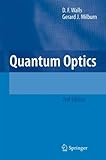# Calculations on Coherent States and Squeezed States (4)Quantum Optics

This is intended to fill some gaps in calculation details in the book above.

#### 5.1.1 Degenerate Parametric Amplifier

##### Preparation: basics of the interaction picture

Suppose that the Hamiltonian consists of the time-independent partplus the time-dependent interaction part.Define the state vector and the interaction term in the interaction picture in conjunction with the ones in the Schroedinger pictureYou can prove that they follow the equation of motion below.To calculate the expectation value for an operator, define the operator in the interaction picture and combine it with the state in the interaction picture.Since the operator in the interaction picture is the same as the one in the Hisenberg picture with, it follows the equation of motion below.In the special case, when the interaction operatorand your operatorsin the interaction picture are time-independent, you have the following relationships.--- (4.1)

Especially, the latter means that the operatorin the Heisenberg picture is obtained as--- (4.2)

Hence it follows the equations of motion below.--- (4.3)

##### Degenerate Parametric AmplifierUsing the relationship (1.1), you can prove that:So this is a special case of the time-independent.

Calculatein the interaction picture.Hence,Now we define the rotated coordinates at a fixed time:Convert it to the interaction picture and set.This means that the (time-independent) operatorsandrepresent the rotated coordinates atin the interaction picture.

Now you can use (4.2) and (4.3) to figure out the rotated coordinates in the Heisenberg picture to understand the physical effects on the initial state.Likewise,Hence,So you can see the squeezing effect on the rotated coordinates.

Sinceare the rotated coordinates in the interaction picture, and they are time-independent, you can apply (4.1) to calculate the expectation value asAnd now you can see that the time evolutionhas the same effect as the squeeze operatorThis explains the squeezing effect obtained above.